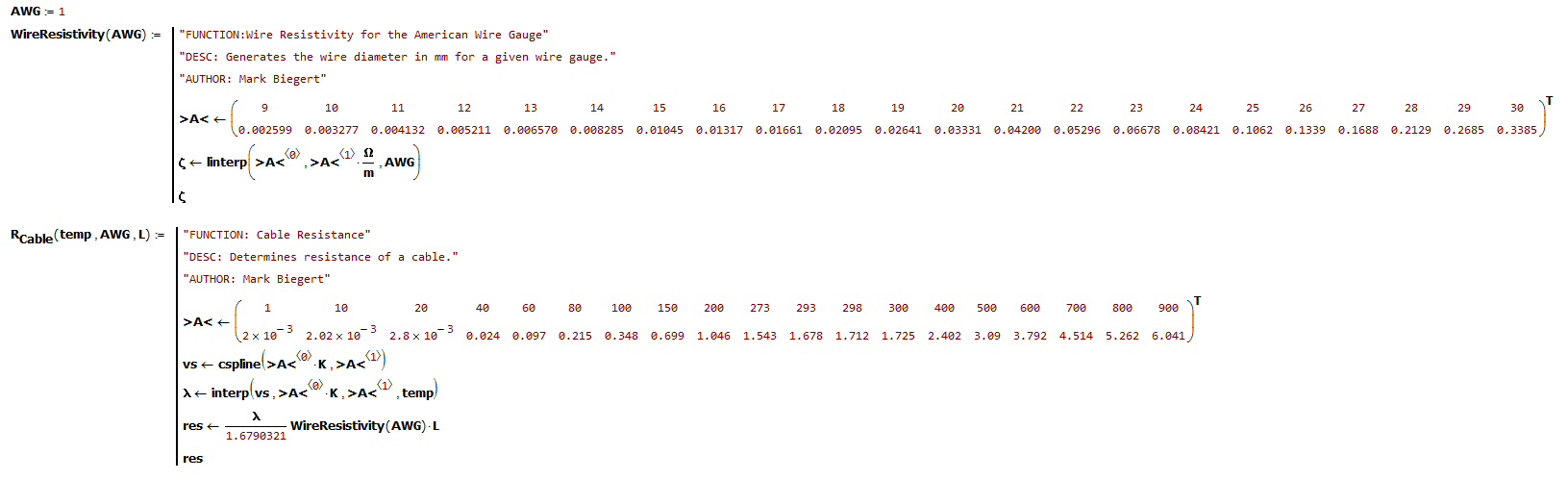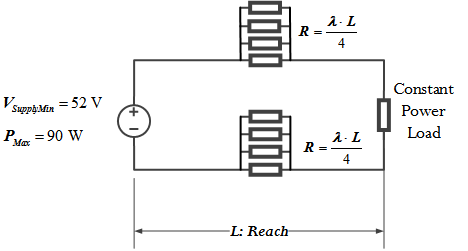# Quick Look at a High-Power PoE Graph

Quote of the Day

Whatever you are, be a good one.

— Abraham Lincoln

## Introduction

I have been sitting in a meeting on a high power version of Power over Ethernet (PoE) known as IEEE 802.3bt. It supports 90 W of output power with a guarantee of 71 W at the load. During the talk, Figure 1 was discussed (my version of the chart). When I am given some mathematical information, I like to experiment with it to see if I understand what I am being told.

In this post, I am going to summarize some quick calculations that I did while sitting in the meeting that helped me understand how this proposed version of PoE transfers power over a data cable. The math shown is simple, but of a type that is very common for a working electrical engineer.

## Background

### Definitions

Power over Ethernet (PoE)
PoE is IEEE 802.3af/at which supports sending power and data over the same category 5e Ethernet cable, which contains four wire-pairs (i.e. 8 wires total). PoE is enormously popular because only one cable is required to network an Ethernet-fed device, which greatly reduces the cost and complexity of networking remote devices, like cameras. For the version of PoE discussed in this post, power is transmitted over two wire-pairs by applying a DC voltage between each pair (see Figure 1). Superimposing DC on the wire-pairs does not interfere with data transmission because Ethernet uses differential signaling.
Type 1 PoE
Type 1 PoE is an IEEE standard (802.3af) for transferring as much as 13 W over an Ethernet cable.
Type 2 PoE
Type 2 PoE is an IEEE standard (802.3at) for transferring as much as 25.5 W over an Ethernet cable. The standard is also known as  "PoE+".
Power Supplying Device (PSE)
A PSE is a device that provides power on an Ethernet cable.
Powered Device (PD)
A PD is a device powered by a PSE.
IEEE 803.3bt
A proposed version of PoE that can transmit as much as 71 W over an Ethernet cable. The extra power is achieved by using all four available wire pairs (8 wires total).

### Post Objective

My objective with this post is to show how a number of important features of the proposed PoE version can be derived from Figure 1.

## Analysis

### Key Graph Characteristics

Figure 1 is a simple graph:

• It is linear with a y-intercept of 90 W (the maximum allowed source power) and a slope of -0.19 W/m.
• The graph ends with a power of 71 W and a range of 100 m, which is the maximum reach guaranteed for 1000Base-T Ethernet.
• The power source have an output voltage that must be greater than 52 V with a load of 90 W.

We also need know that standard Category 5e (Cat 5e) Ethernet cable is composed of 4 pairs of 24 American Wire Gauge (AWG) wire.

### Calculations

#### Wire Resistance Utility Functions

Figure 2 shows the utility functions that I use to compute the resistance of annealed copper wire as a function of temperature, length, and gauge. I have used these functions for years  – they are based on curve fits of old Bellcore wire resistance data.Figure 2: Utility Functions for Computing Wire Resistance as a Function of Temperature, Length, and Wire Gauge.

#### Resistance Model

Figure 3 shows the circuit model for this PoE circuit.Figure 3: PoE Resistance Model. L is the total length of the wire, R is the total resistance of the wire, and λ is the 2-way resistance of the wire.

#### Maximum Supply Current (IMax) and Line Resistance Per Meter (λ)

Figure 4 shows how to use Ohm's law with Figure 1 to determine (a) the maximum current on the line, and (b) the two-way resistance of the line per meter, and (c) the wire gauge that meets this resistance requirement. As you would expect, 24 AWG wire meets the requirements for the PoE standard and is the wire gauge used in Cat 5e cable.

### Minimum Load Voltage

Figure 5 shows how we can compute the load voltage when the cable is carrying the maximum current over the maximum length cable.

This is the minimum voltage at which the load's power converter must operate.

### Cross-Check Calculations

Figure 6  shows some calculations that demonstrate that my results are internally consistent.

## Conclusion

I have always felt that the today's PoE power limits of 15.5 W (type 1) and 25.5 W (type 2) are not adequate for many applications involving MIMO wireless access points. This new standard will be a real boon to customers with wireless coverage issues.

Save

This entry was posted in Electronics, Power Over Ethernet. Bookmark the permalink.